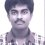# Help: Functional Equation 3

$f\left( x \right) f\left( y \right) =f\left( x \right) +f\left( y \right) +f\left( xy \right) -2$

If $f\left( x \right)$ is a polynomial satisfying the equation above for all $x$ and $y$ and $f\left( 2 \right)=5$, then compute $\displaystyle \lim _{ x\rightarrow 2 }{ f^{ ' }\left( x \right) }$.

If anyone solves this one, please do tell me what is the error in this one5 years, 2 months ago

This discussion board is a place to discuss our Daily Challenges and the math and science related to those challenges. Explanations are more than just a solution — they should explain the steps and thinking strategies that you used to obtain the solution. Comments should further the discussion of math and science.

When posting on Brilliant:

• Use the emojis to react to an explanation, whether you're congratulating a job well done , or just really confused .
• Ask specific questions about the challenge or the steps in somebody's explanation. Well-posed questions can add a lot to the discussion, but posting "I don't understand!" doesn't help anyone.
• Try to contribute something new to the discussion, whether it is an extension, generalization or other idea related to the challenge.

MarkdownAppears as
*italics* or _italics_ italics
**bold** or __bold__ bold
- bulleted- list
• bulleted
• list
1. numbered2. list
1. numbered
2. list
Note: you must add a full line of space before and after lists for them to show up correctly
paragraph 1paragraph 2

paragraph 1

paragraph 2

[example link](https://brilliant.org)example link
> This is a quote
This is a quote
    # I indented these lines
# 4 spaces, and now they show
# up as a code block.

print "hello world"
# I indented these lines
# 4 spaces, and now they show
# up as a code block.

print "hello world"
MathAppears as
Remember to wrap math in $$ ... $$ or $ ... $ to ensure proper formatting.
2 \times 3 $2 \times 3$
2^{34} $2^{34}$
a_{i-1} $a_{i-1}$
\frac{2}{3} $\frac{2}{3}$
\sqrt{2} $\sqrt{2}$
\sum_{i=1}^3 $\sum_{i=1}^3$
\sin \theta $\sin \theta$
\boxed{123} $\boxed{123}$

Sort by:

Hey there!
We have,
$f(x)f(y)-f(x)-f(y)+1\quad =\quad f(xy)-1\\ (f(x)-1)(f(y)-1)\quad =\quad f(xy)-1\quad \\ g(x)=f(x)-1 \\ \Rightarrow g(x)g(y) = g(xy) \\$

This is famous, see here.
We then have that,
$g(x) = x ^ t, t = 2. \\ g(x) = x^2 \\ f(x) = x^2 + 1 \\ f'(x) = 2x \\ \Rightarrow \lim _{ x\rightarrow 2 }{ f^{ ' }(x) } = 4$

- 5 years, 2 months ago

Put x and y as 2 which will give you the value of f(4) as 17

Put x as 4 and y as 2 and you get f(8) as 65. Similarly you can check with other values and this leads to the conclusion that f(x) is one greater than the square of x.

Hence the limit computation will be 4

Please excuse me if you were looking for a formal proof or evaluation of the same.

- 5 years, 2 months ago

- 5 years, 2 months ago

@Rishabh Cool, this question again.

- 5 years, 2 months ago